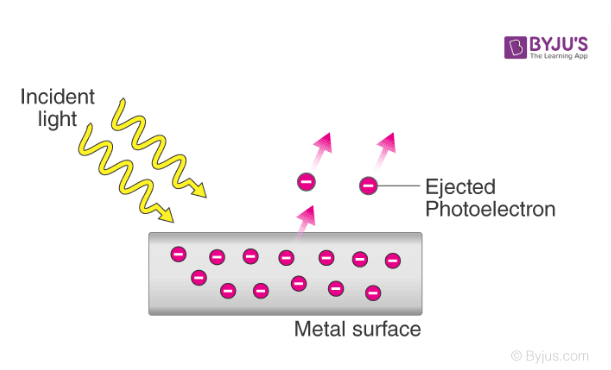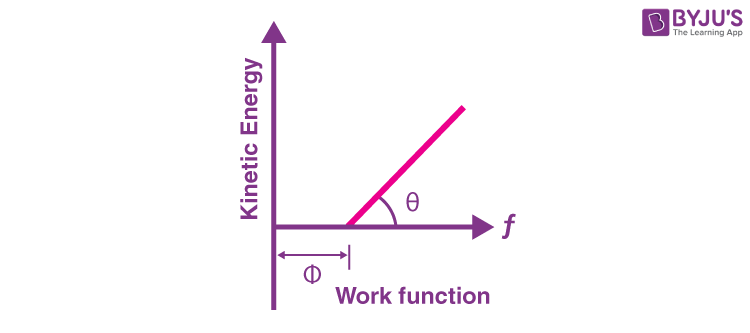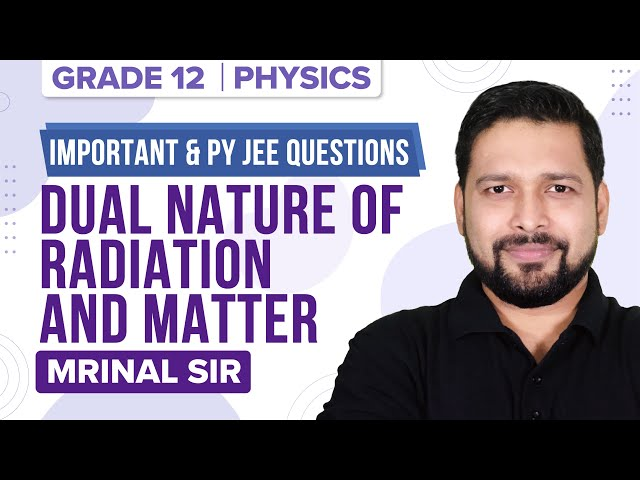Know more about the Repeaters Batch Know more about the Repeaters Batch

# JEE Main Photoelectric Effect Previous Year Questions with Solutions

The phenomenon of the photoelectric effect was discovered by Heinrich Hertz in 1887. When light rays (electromagnetic radiation) of suitable frequency are incident on the surface of the metal, electrons are ejected from the surface. This phenomenon is called the photoelectric effect. The electrons liberated from the surface are called photoelectrons. The current due to the photoelectric effect is called photoelectric current or photocurrent. In the photoelectric effect, light energy is converted into electrical energy. The process through which photoelectrons are ejected from the surface of the metal due to the action of light is commonly referred to as photoemission.

It is found that different substances respond to electromagnetic radiations of different frequencies. An illustration detailing the emission of photoelectrons as a result of the photoelectric effect is provided below.## JEE Main Previous Year Solved Questions on Photoelectric Effect

Q1: Surface of a certain metal is first illuminated with light of wavelength λ1 = 350 nm and then by the light of wavelength λ2 = 540 nm. It is found that the maximum speed of the photoelectrons in the two cases differ by a factor of 2. The work function of the metal (in eV) is close to (Energy of photon = (1240/ λ) eV)

(a) 5.6

(b) 2.5

(c) 1.4

(d) 1.8

Solution

Using Einstein photoelectric equation, E – Φ = (½)mv2

E1 = Φ + (½) (2v)2

E2 = Φ + (½) (v)2

⇒ (E1 – Φ)/(E2 – Φ) = 4

(hc/λ1– Φ)/(hc/λ2– Φ) = 4

(hc/λ1– Φ) = 4(hc/λ2– Φ)

Φ = (hc/3)(4/λ2 – 1/λ1) = (1240/3)[(4 x 350 – 540)/(350 x 540)] ≈1.88 eV

Q2: When a certain photosensitive surface is illuminated with monochromatic light of frequency f, the stopping potential for the photocurrent is (-V0/2). When the surface is illuminated by monochromatic light of frequency f/2, the stopping potential is –V0. The threshold frequency for photoelectric emission is

(a) 4f/3

(b) 2f

(c) 5f/3

(d) 3f/2

Solution

hf = Φ + (v0/2)e———-(1)

hf/2 = Φ + v0e————(2)

(½) = (hf – Φ)/( ((hf/2) – Φ)

hf0 = (3/2)hf (since Φ = hf0 )

f0 = (3/2)f

Q3: In a photoelectric effect experiment the threshold wavelength of light is 380 nm. If the wavelength of incident light is 260 nm, the maximum kinetic energy of emitted electrons will be given E (in eV) = [1237/λ(in nm)]

(a) 15.1 eV

(b) 4.5 eV

(c) 1.5 eV

(d) 3.0 eV

Solution

Given: λ0 = 380 nm, λi = 260 nm

Kmax = h (fi – f0)

= h[(c/λi) – (c/λ0)] = hc[(λ0– λi)/(λ0λi)]

= 1237[(380 – 260)/(380 x 260)] eV = 1.5 eV

Q4: Photon of frequency has a momentum associated with it. If c is the velocity of light, the momentum is

(a) hf/c

(b) f/c

(c) hfc

(d) hf/c2

Solution

The energy of a photon E = hf… (1)

Also E = pc… (2)

where p is the momentum of a photon

From (1) and (2) we get hf = pc

p = hf/c

Q5: The time by a photoelectron to come out after the photon strikes is approximately

(a) 10–1 s

(b) 10–4 s

(c) 10–10 s

(d) 10–16 s

Solution

Emission of photo-electron starts from the surface after the incidence of photons in about 10–10 sec

Q6: The work function of a substance is 4.0 eV. The longest wavelength of light that can cause photoelectron emission from this substance is approximately

(a) 540 nm

(b) 400 nm

(c) 310 nm

(d) 220 nm

Solution

Let λm = Longest wavelength of light

(hc/λm) = Φ (work function)

λm= hc/Φ = (6.63 x 10-34) x (3 x108)/(4.0 x1.6 x10-19) = 310 nm

Q7: According to Einstein’s photoelectric equation, the plot of the kinetic energy of the emitted photoelectrons from a metal vs the frequency of the incident radiation gives a straight line whose slope

(a) depends on the nature of the metal used

(b) depends on the intensity of the radiation

(c) depends both on the intensity of the radiation and the metal used

(d) is the same for all metals and independent of the intensity of the radiation.

Solution

According to Einstein’s equation, Kinetic energy = hf – Φ where kinetic energy and f (frequency) are variables, compare it with the equation, y = mx + cTherefore, the slope of line = h, h is Planck’s constant.

Hence, the slope is the same for all metals and independent of the intensity of radiation.

Answer : (d) is the same for all metals and independent of the intensity of the radiation

Q8: This question has Statement-1 and Statement-2. Of the four choices given after the statements, choose the one that best describes the two statements.

Statement-1: When ultraviolet light is incident on a photocell, its stopping potential is V0 and the maximum kinetic energy of the photoelectrons is Kmax. When the ultraviolet light is replaced by X-rays, both V0 and Kmax increase.

Statement-2: Photoelectrons are emitted with speeds ranging from zero to a maximum value because of the range of frequencies present in the incident light.

(a) Statement-1 is true, Statement-2 is false

(b) Statement-1 is true, Statement-2 is True; Statement-2 is the correct explanation of Statement-1

(c) Statement-1 is true, Statement-2 is True; Statement-2 is not the correct explanation of Statement-1

(d) Statement-1 is False, Statement-2 is true

Solution

According to Einstein’s photoelectric equation

Kmax = hf – Φ0

where, f = frequency of incident light

Φ0 = work function of the metal

Since the frequency of ultraviolet light is less than the frequency of x-rays, the energy of the photon will be more than x-rays

Since Kmax = eV0

V0 = (hf/e) – (Φ0/e)

As fX-rays > fUltraviolet

Therefore, both Kmax and V0 increase when ultraviolet light is replaced by X-rays.

Statement-2 is false.

Answer: (a) Statement-1 is true, Statement-2 is false

Q9: The maximum velocity of the photoelectrons emitted from the surface is v when the light of frequency n falls on a metal surface. If the incident frequency is increased to 3n, the maximum velocity of the ejected photoelectrons will be

(a) more than √3v

(b) less than √3v

(c) v

(d) equal to √3v

Solution

E1 = (½) mv2 = hn – Φ

If incident frequency is increased to 3n

E2 = 1/2 mv’2 = 3hn – Φ = 3(hn – Φ ) + 2Φ = 3 × (½) mv2 + 2Φ

v’2 = 3v2 + 4Φ/m; v’ > v√3

Q10: In a Frank-Hertz experiment, an electron of energy 5.6 eV passes through mercury vapour and emerges with an energy 0.7 eV. The minimum wavelength of photons emitted by mercury atoms is close to

(a) 220 nm

(b) 1700 nm

(c) 250 nm

(d) 2020 nm

Solution

The minimum wavelength of emitted photons is

(hc/∆E) = (1240)/(5.6 – 0.7) = 250 nm